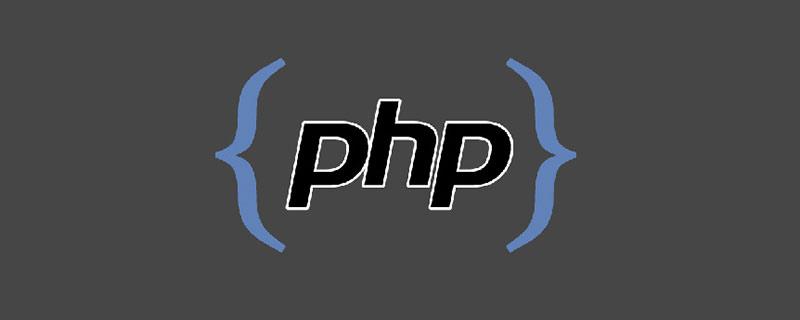# php final 方法怎么用_亿码酷站_编程开发技术教程

【亿码酷站-编程开发教程】收集全网优质教程及源码资源！PHP 面向对象 final类与final方法

final—用于类、方法前。

final类—不可被继承。

final方法—不可被覆盖。

```<?
//声明一个final类Math
final class Math
{
public static \$pi = 3.14;
public function __toString()
{
return "这是Math类。";
}
}
\$math = new Math();
echo \$math;
//声明类SuperMath 继承自 Math类
class SuperMath extends Math
{
}
//执行会出错，final类不能被继承。
?>```

`Fatal error: Class SuperMath may not inherit from final class (Math) in E:\PHPProjects\test.php on line 16`

```<?
//声明一个final类Math
class Math
{
public static \$pi = 3.14;
public function __toString()
{
return "这是Math类。";
}
public final function max(\$a,\$b)
{
return \$a > \$b ? \$a : \$b ;
}
}
//声明类SuperMath 继承自 Math类
class SuperMath extends Math
{
public final function max(\$a,\$b){}
}
//执行会出错，final方法不能被重写。
?>```

`Fatal error: Class SuperMath may not inherit from final class (Math) in E:\PHPProjects\test.php on line 16`

php final 方法怎么用

php动态修改网页title的方法

php 字符串怎么转八进制数

php怎么将字符串转为浮点型

## php如何隐藏手机号_编程技术_亿码酷站

php如何隐藏手机号_编程技术_亿码酷站

php浏览器怎么显示错误提示